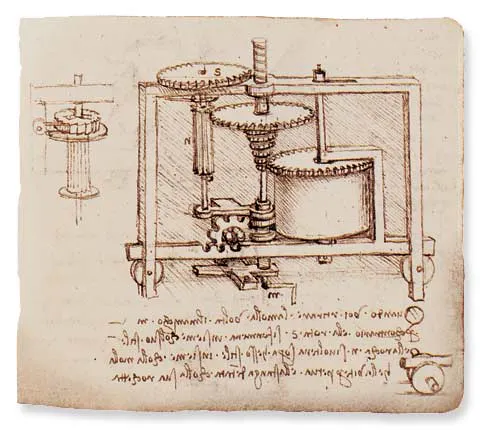Published

Ubidots Signal Recorder

This project gives the code to assemble a 4 channel discrete signal recorder, the board is a LinkIt one.

IntermediateProtip1 hour315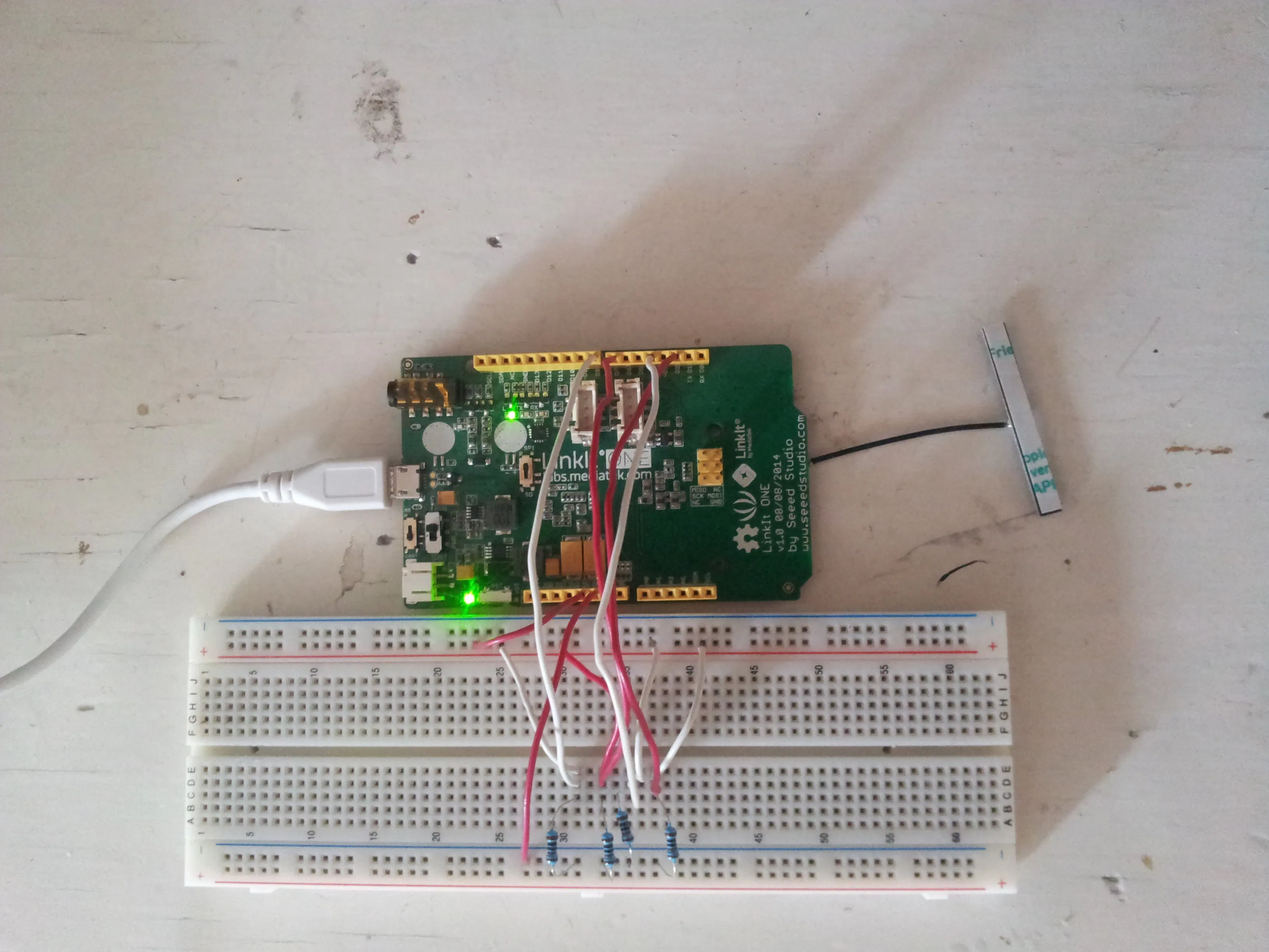Things used in this project

Hardware components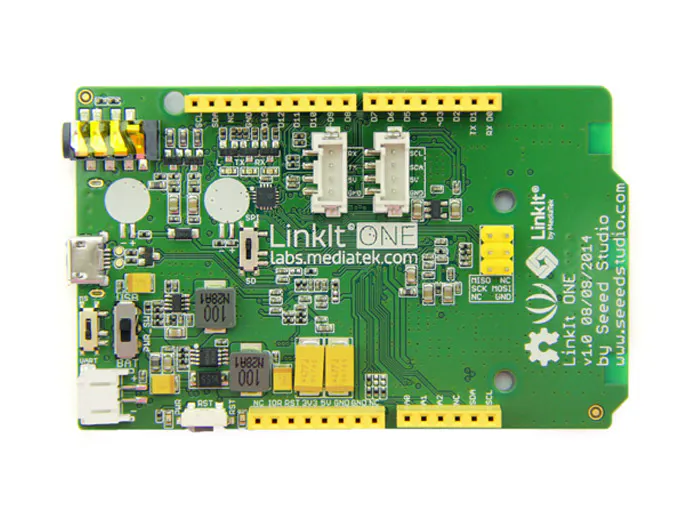×1Resistor 1k ohm
×4Jumper wires (generic)
×1

Software apps and online servicesArduino IDEUbidots

Schematics

Ubidots work

1. create an account
2. in sources create a new data source
3. copy the label of your device and paste into the code
4. from API credentials copy the token and paste into the code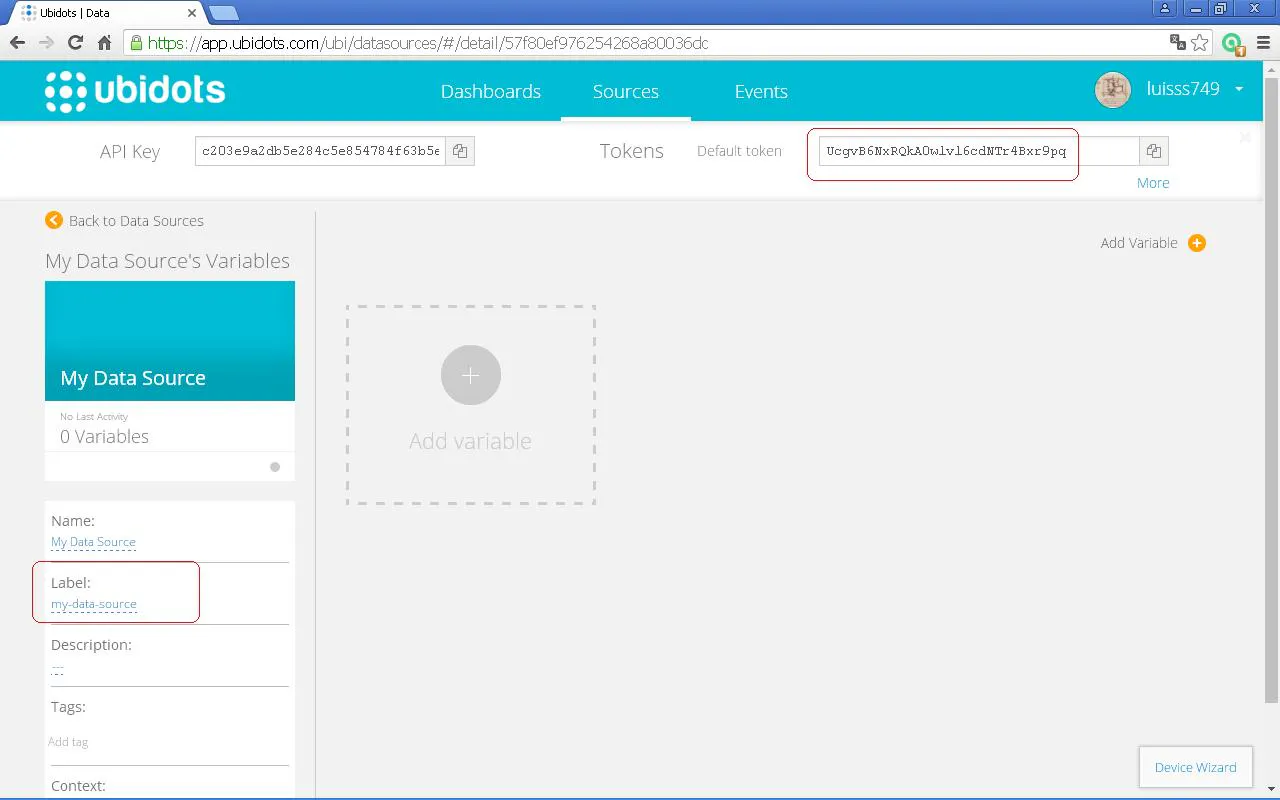Check the operation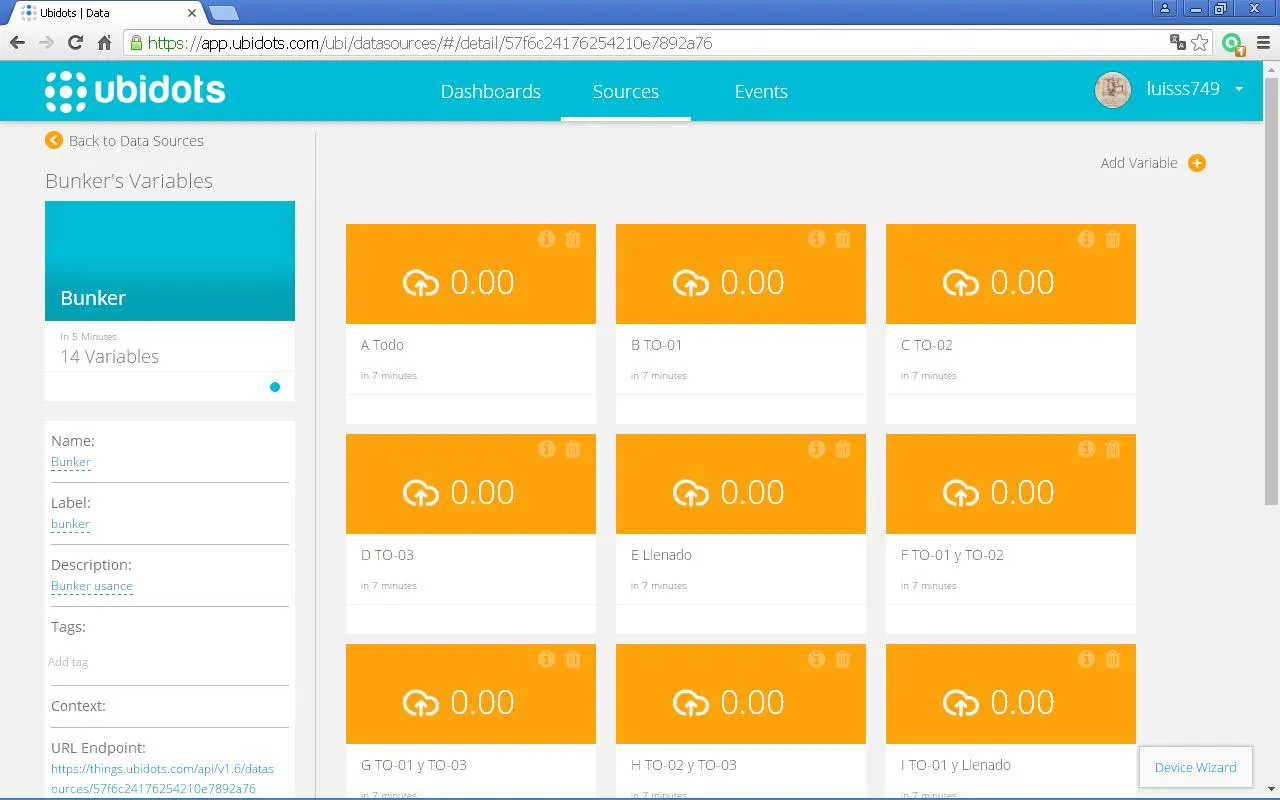Code

Ubidots discrete signal recorder

Arduino
1.- connect a pull down resistor-switch array on input pins 2 4 7 y 8
2. change the line 9 and 10 of with your wifi AP
3. from ubidots copy and paste the token and the label of your device in lines 7 and 8
3.-send the code to the board
4. check the operation in serial monitor
```#include <LWiFi.h>
#include <LWiFiClient.h>
#include <PubSubClient.h>

// These are the variables you will want to change based on your IOT data streaming account / provider

#define TOPIC "/v1.6/devices/YOURDEVICELABEL"
#define TOKEN "YOURUBIDOTSTOKEN"
#define WIFI_AP ""
#define WIFI_AUTH LWIFI_WPA
char payload;  // Reserve a char to store the Ubidots data. Account for 60 bytes per variable.
char le;
char mqttBroker[] = "things.ubidots.com";
String response;
LWiFiClient c;
PubSubClient client(c);

void reconnect() {
// Loop until we're reconnected
while (!client.connected()) {
Serial.print("Attempting MQTT connection...");
// Attempt to connect
Serial.println("connected");
} else {
Serial.print("failed, rc=");
Serial.print(client.state());
Serial.println(" try again in 5 seconds");
// Wait 5 seconds before retrying
delay(5000);
}
}
}

int PB1 = 2;
int PB2 = 4;
int PB3 = 7;
int PB4 = 8;

int A = 0;
int B = 0;
int C = 0;
int D = 0;
int E = 0;
int F = 0;
int G = 0;
int H = 0;
int I = 0;
int J = 0;
int K = 0;
int L = 0;
int M = 0;
int N = 0;

void setup()
{
Serial.begin(115200);             // setup Serial port

LWiFi.begin();
// keep retrying until connected to AP
Serial.println("Connecting to AP");
{
delay(1000);
}
Serial.println("Wifi connected!");
client.setServer(mqttBroker, 1883);

pinMode(PB1, INPUT);
pinMode(PB2, INPUT);
pinMode(PB3, INPUT);
pinMode(PB4, INPUT);

}

void loop()
{

if (TO01 ==  1 && TO02 == 1 && TO03 == 1 && Llenado == 1)
{
A = 100;
}
else
{
A = 0;
}

if (TO01 == 1 && A == 0 && F == 0 && G == 0 && I == 0 && L == 0 && M == 0)
{
B = 100;
}
else
{
B = 0;
}
if (TO02 == 1 && A == 0 && F == 0 && H == 0 && J == 0 && L == 0 && N == 0)
{
C = 100;
}
else
{
C = 0;
}
if (TO03 == 1 && A == 0 && G == 0 && H == 0 && K == 0 && M == 0 && N == 0)
{
D = 100;
}
else
{
D = 0;
}
if (Llenado == 1 && A == 0 && I == 0 && J == 0 && K == 0 && L == 0 && M == 0 && N == 0)
{
E = 100;
}
else
{
E = 0;
}
if (TO01 == 1 && TO02 == 1 && A == 0 && L == 0)
{
F = 100;
}
else
{
F = 0;
}
if (TO01 == 1 && TO03 == 1 && A == 0 && M == 0)
{
G = 100;
}
else
{
G = 0;
}
if (TO02 == 1 && TO03 == 1 && A == 0 && N == 0)
{
H = 100;
}
else
{
H = 0;
}
if (TO01 == 1 && Llenado == 1 && A == 0 && L == 0 && M == 0)
{
I = 100;
}
else
{
I = 0;
}
if (TO02 == 1 && Llenado == 1 && A == 0 && L == 0 && N == 0)
{
J = 100;
}
else
{
J = 0;
}
if (TO03 == 1 && Llenado == 1 && A == 0 && M == 0 && N == 0)
{
K = 100;
}
else
{
K = 0;
}
if (TO01 == 1 && TO02 == 1 && Llenado == 1 && A == 0)
{
L = 100;
}
else
{
L = 0;
}
if (TO01 == 1 && TO03 == 1 && Llenado == 1 && A == 0)
{
M = 100;
}
else
{
M = 0;
}
if (TO02 == 1 && TO03 == 1 && Llenado == 1 && A == 0)
{
N = 100;
}
else
{
N = 0;
}

if (!client.connected()) {
reconnect();
}
client.loop();

delay(1000);
}
```

Credits

Luis Salazar

1 project • 5 followers
Mechanical Engineer Electronics hobbyst
Thanks to AgustinP.